### Home > CAAC > Chapter 14 > Lesson 14.3.1.6 > Problem3-78

3-78.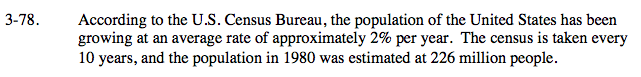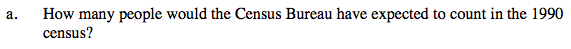Use the multiplier and the year 1980 as year 0 to write an equation.

226(1.02)10 = 275.5 million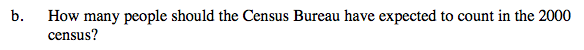See part (a).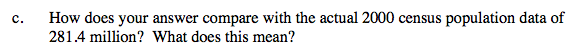How great is the error? Is the population growth faster or slower than expected?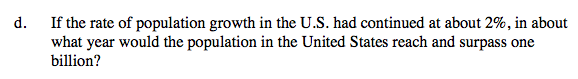How many million is one billion? Substitute this into the equation you wrote in part (a).

1000 = 226(1.02)x
4.42 = (1.02)x

2055

You will need to guess and check to figure out x.